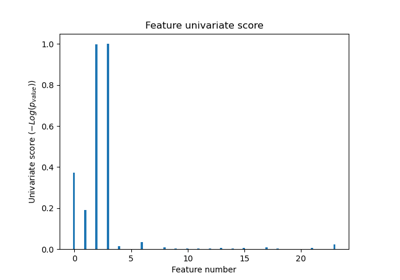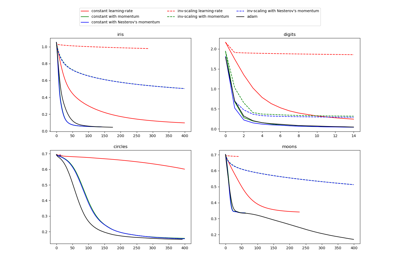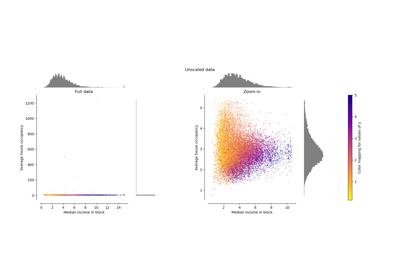# sklearn.preprocessing.MinMaxScaler¶

class sklearn.preprocessing.MinMaxScaler(feature_range=0, 1, *, copy=True, clip=False)[source]

Transform features by scaling each feature to a given range.

This estimator scales and translates each feature individually such that it is in the given range on the training set, e.g. between zero and one.

The transformation is given by:

X_std = (X - X.min(axis=0)) / (X.max(axis=0) - X.min(axis=0))
X_scaled = X_std * (max - min) + min


where min, max = feature_range.

This transformation is often used as an alternative to zero mean, unit variance scaling.

Read more in the User Guide.

Parameters
feature_rangetuple (min, max), default=(0, 1)

Desired range of transformed data.

copybool, default=True

Set to False to perform inplace row normalization and avoid a copy (if the input is already a numpy array).

clip: bool, default=False

Set to True to clip transformed values of held-out data to provided feature range.

Attributes
min_ndarray of shape (n_features,)

Per feature adjustment for minimum. Equivalent to min - X.min(axis=0) * self.scale_

scale_ndarray of shape (n_features,)

Per feature relative scaling of the data. Equivalent to (max - min) / (X.max(axis=0) - X.min(axis=0))

New in version 0.17: scale_ attribute.

data_min_ndarray of shape (n_features,)

Per feature minimum seen in the data

New in version 0.17: data_min_

data_max_ndarray of shape (n_features,)

Per feature maximum seen in the data

New in version 0.17: data_max_

data_range_ndarray of shape (n_features,)

Per feature range (data_max_ - data_min_) seen in the data

New in version 0.17: data_range_

n_samples_seen_int

The number of samples processed by the estimator. It will be reset on new calls to fit, but increments across partial_fit calls.

minmax_scale

Equivalent function without the estimator API.

Notes

NaNs are treated as missing values: disregarded in fit, and maintained in transform.

For a comparison of the different scalers, transformers, and normalizers, see examples/preprocessing/plot_all_scaling.py.

Examples

>>> from sklearn.preprocessing import MinMaxScaler
>>> data = [[-1, 2], [-0.5, 6], [0, 10], [1, 18]]
>>> scaler = MinMaxScaler()
>>> print(scaler.fit(data))
MinMaxScaler()
>>> print(scaler.data_max_)
[ 1. 18.]
>>> print(scaler.transform(data))
[[0.   0.  ]
[0.25 0.25]
[0.5  0.5 ]
[1.   1.  ]]
>>> print(scaler.transform([[2, 2]]))
[[1.5 0. ]]


Methods

 fit(X[, y]) Compute the minimum and maximum to be used for later scaling. fit_transform(X[, y]) Fit to data, then transform it. get_params([deep]) Get parameters for this estimator. Undo the scaling of X according to feature_range. partial_fit(X[, y]) Online computation of min and max on X for later scaling. set_params(**params) Set the parameters of this estimator. Scale features of X according to feature_range.
fit(X, y=None)[source]

Compute the minimum and maximum to be used for later scaling.

Parameters
Xarray-like of shape (n_samples, n_features)

The data used to compute the per-feature minimum and maximum used for later scaling along the features axis.

yNone

Ignored.

Returns
selfobject

Fitted scaler.

fit_transform(X, y=None, **fit_params)[source]

Fit to data, then transform it.

Fits transformer to X and y with optional parameters fit_params and returns a transformed version of X.

Parameters
X{array-like, sparse matrix, dataframe} of shape (n_samples, n_features)

Input samples.

yndarray of shape (n_samples,), default=None

Target values (None for unsupervised transformations).

**fit_paramsdict

Returns
X_newndarray array of shape (n_samples, n_features_new)

Transformed array.

get_params(deep=True)[source]

Get parameters for this estimator.

Parameters
deepbool, default=True

If True, will return the parameters for this estimator and contained subobjects that are estimators.

Returns
paramsmapping of string to any

Parameter names mapped to their values.

inverse_transform(X)[source]

Undo the scaling of X according to feature_range.

Parameters
Xarray-like of shape (n_samples, n_features)

Input data that will be transformed. It cannot be sparse.

Returns
Xtndarray of shape (n_samples, n_features)

Transformed data.

partial_fit(X, y=None)[source]

Online computation of min and max on X for later scaling.

All of X is processed as a single batch. This is intended for cases when fit is not feasible due to very large number of n_samples or because X is read from a continuous stream.

Parameters
Xarray-like of shape (n_samples, n_features)

The data used to compute the mean and standard deviation used for later scaling along the features axis.

yNone

Ignored.

Returns
selfobject

Fitted scaler.

set_params(**params)[source]

Set the parameters of this estimator.

The method works on simple estimators as well as on nested objects (such as pipelines). The latter have parameters of the form <component>__<parameter> so that it’s possible to update each component of a nested object.

Parameters
**paramsdict

Estimator parameters.

Returns
selfobject

Estimator instance.

transform(X)[source]

Scale features of X according to feature_range.

Parameters
Xarray-like of shape (n_samples, n_features)

Input data that will be transformed.

Returns
Xtndarray of shape (n_samples, n_features)

Transformed data.

## Examples using sklearn.preprocessing.MinMaxScaler¶